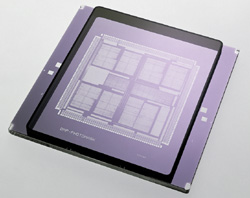# Math Modeling in Indudustry Team 3

Speaker: Apo Sezginer

Date: Tue, Aug 5, 2014

Location: PIMS, University of British Columbia

Conference: IMA-PIMS Math Modeling in Industry Workshop

Subject:

Class: Scientific

### Abstract:

Integrated circuits are manufactured by optical projection lithography.  The circuit pattern is etched on a master copy, the photomask.  Light is projected through the photomask and its image is formed on the semiconductor wafer under production.  The image is transferred to the integrated circuit by a photographic process.  On the order of 40 lithography steps are needed to produce an integrated circuit.   Most advanced lithography is performed using the 193 nm ArF excimer wavelength, about three times smaller than the wavelength of visible red light.  Critical dimensions of the circuit pattern are smaller than the wavelength of the projected light.  Sub-wavelength resolution is achieved by optical resolution enhancement techniques and the non-linearity of the chemistry.

Calculating the optical image accurately and rapidly is required for two reasons: first the design of the photomask is an inverse problem.  A good forward solution is needed to solve the inverse problem iteratively.  Second, the photomask is inspected by a microscope to find manufacturing defects.  The correct microscope image is calculated, and the actual microscope image is compared to the calculated reference image to find defects.  The most significant part of the image calculation is the diffraction of the illuminating wave by the photomask.  Although rigorous solution of Maxwell's Equations by numerical methods is well known, either the speed or the accuracy of known methods is not satisfactory.  The most commonly used method is Kirchhoff approximation amended by some fudge factors to make it closer to the rigorous solution.

Kirchhoff solved the problem diffraction of light through an arbitrarily shaped aperture in an opaque screen at the end of 19th century.  He had a very practical approximation for the near-field of the screen, on the side that is opposite to the light source.  At a point on the screen, he ignored that there is an aperture.  At a point at the aperture, he ignored that there is a screen.  He used Green's theorem to propagate this estimate of the near-field to the far-field.  Kirchhoff’s near-field approximation is accurate for points that are a few wave-lengths away from the edges.   The Kirchhoff near-field is discontinuous at the edges and it violates boundary conditions for Maxwell’s Equations.  To this day an amended form of Kirchhoff’s approximation provides the best known accuracy-speed trade-off to calculate the image of a photomask.The Goal of this Project

We will attempt to improve the accuracy of the Kirchhoff’s approximation.  We will cast Maxwell’s Equations into a linear matrix equation Ax=b where x is a vector of electric and magnetic field values.  This can be done either using finite differences or using a weak (integral) form of Maxwell’s Equations.  We will initialize the vector x with the Kirchhoff solution.  We will use an iterative linear equation solver such as GMRES.  The goal is to improve the solution in very few iterations.